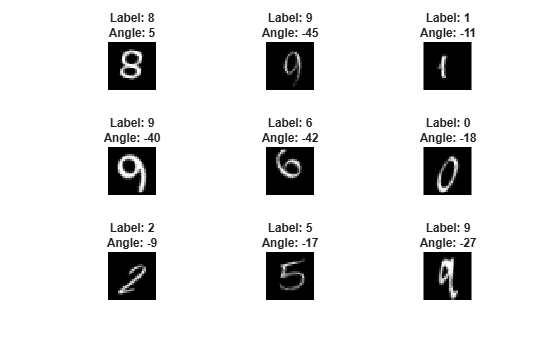# Make Predictions Using `dlnetwork` Object

This example shows how to make predictions using a `dlnetwork` object by splitting data into mini-batches.

For large data sets, or when predicting on hardware with limited memory, make predictions by splitting the data into mini-batches. When making predictions with `SeriesNetwork` or `DAGNetwork` objects, the `predict` function automatically splits the input data into mini-batches. For `dlnetwork` objects, you must split the data into mini-batches manually.

### Load `dlnetwork` Object

Load a trained `dlnetwork` object and the corresponding classes.

```s = load("digitsCustom.mat"); dlnet = s.dlnet; classes = s.classes;```

Load the digits data for prediction.

```digitDatasetPath = fullfile(matlabroot,'toolbox','nnet','nndemos', ... 'nndatasets','DigitDataset'); imds = imageDatastore(digitDatasetPath, ... 'IncludeSubfolders',true);```

### Make Predictions

Loop over the mini-batches of the test data and make predictions using a custom prediction loop.

Use `minibatchqueue` to process and manage the mini-batches of images. Specify a mini-batch size of 128. Set the read size property of the image datastore to the mini-batch size.

For each mini-batch:

• Use the custom mini-batch preprocessing function `preprocessMiniBatch` (defined at the end of this example) to concatenate the data into a batch and normalize the images.

• Format the images with the dimensions `'SSCB'` (spatial, spatial, channel, batch). By default, the `minibatchqueue` object converts the data to `dlarray` objects with underlying type `single`.

• Make predictions on a GPU if one is available. By default, the `minibatchqueue` object converts the output to a `gpuArray` if a GPU is available. Using a GPU requires Parallel Computing Toolbox™ and a CUDA® enabled NVIDIA® GPU with compute capability 3.0 or higher.

```miniBatchSize = 128; imds.ReadSize = miniBatchSize; mbq = minibatchqueue(imds,... "MiniBatchSize",miniBatchSize,... "MiniBatchFcn", @preprocessMiniBatch,... "MiniBatchFormat","SSCB");```

Loop over the minibatches of data and make predictions using the `predict` function. Use the `onehotdecode` function to determing the class labels. Store the predicted class labels.

```numObservations = numel(imds.Files); YPred = strings(1,numObservations); predictions = []; % Loop over mini-batches. while hasdata(mbq) % Read mini-batch of data. dlX = next(mbq); % Make predictions using the predict function. dlYPred = predict(dlnet,dlX); % Determine corresponding classes. predBatch = onehotdecode(dlYPred,classes,1); predictions = [predictions predBatch]; end```

Visualize some of the predictions.

```idx = randperm(numObservations,9); figure for i = 1:9 subplot(3,3,i) I = imread(imds.Files{idx(i)}); label = predictions(idx(i)); imshow(I) title("Label: " + string(label)) end```### Mini-Batch Preprocessing Function

The `preprocessMiniBatch` function preprocesses the data using the following steps:

1. Extract the data from the incoming cell array and concatenate into a numeric array. Concatenating over the fourth dimension adds a third dimension to each image, to be used as a singleton channel dimension.

2. Normalize the pixel values between `0` and `1`.

```function X = preprocessMiniBatch(data) % Extract image data from cell and concatenate X = cat(4,data{:}); % Normalize the images. X = X/255; end```